## SALICRU, Tecnocampus and UOC cooperates in training engineers specialized in Business Digital Transformation within the Master degree in Industry 4.0

The first edition of the Master’s degree in Industry 4.0 started in the academic year 2017-18. It is jointly organized by the Computing, Multimedia and Telecommunications Studies (EIMT) of the Catalonia Open University (UOC) and the TecnoCampus Polytechnic College, ESUPT, affiliated center to Pompeu Fabra University.It is a non-official on-line two-year master’s course developed over the UOC’s online teaching platform. The course contents are organized into four areas of 12 ECTS each (Cyberphysic Systems Development, Cyberphysic Systems Integration, Smart Manufacturing and Business Digital Transformation). They deeply deal, in depth and based on real case study, with the different technologies and business transformation features that are critical in Industry 4.0, with the aim of providing an integrating training.

In this context, SALICRU has cooperated in the development of the specialization in Business Digital Transformation by providing a study case based on their business model and the needs and challenges faced by a company that designs, manufactures and keeps products which guarantee continuous power supply to their customers.

The case prepared by SALICRU is based on strategic goals for both, product and service, and it takes into account the different disciplines applied in the design of their products. It provides a functional scheme as well as the demands of the production system and the characteristics of the market.

The case raised by SALICRU does not exactly shows neither the company’s reality nor the actual SALICRU’s actual infrastructure, but allows it allows to present a real industry-based to students. Thus, students can work on problems of the real industry and discuss and propose solutions on multidimensional problems: strategy, business, digitalization, innovation, computer security, business intelligence and data analytics.

SALICRU’s case is the basis upon which the resolution of practical issues in each of the modules of the specialization: Management of Digital Transformation, Innovation Management, Cybersecurity Management and Data Analytics, is proposed.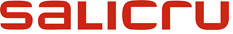The participation of SALICRU in the Master’s degree in Industry 4.0 is an example of the company’s commitment to digital transformation, as Jordi Montero, SALICRU’s R&D manager, comments: The participation of SALICRU in this Master’s degree in Industry 4.0 allows us to achieve a double objective: to collaborate in the training of engineers  specialized in the application of Industry 4.0 and, at the same time, to be able to collect innovative proposals about the business model and the way to work with a product and a market like ours. It is a three-way cooperation in which everyone wins: company, university and students.

According to Dr. Jordi Ayza, responsible teacher of the specialization of Business Digital Transformation: Being able to work on a practical case proposed by a company like SALICRU, which has raised the challenge of Industry 4.0 and is following the path of digital transformation, allows us to show Master’s students a real case, with real problems and with all the complexity of a company with advanced technology, with a globalized production environment and with a plural and international market.

On the other hand, Dr. Pere Tuset, teacher responsible for the Master’s degree in Industry 4.0 by the UOC, highlights the benefits of collaboration between the three entities: At the UOC, leading university in online higher education, we understood that offering this industry-focused master’s degree, which requires working a broad set of disciplines and, at the same time, with a team of professionals with real experience in industry, required working in cooperation with other entities. The organization of this Master’s degree together with the ESUPT and with companies such as SALICRU allows us to offer, as we wanted, the best Master’s degree in Industry 4.0 of the market.

SALICRU researches and develops power electronic devices to guarantee a reliable, clean and continuous power supply, and it is the undisputed leading company in the sector within the Spanish market. https://www.salicru.com/en/## Coverage using network of streets

Tracing a path through a streets’ network from a starting point to calculate the coverage along the network, it’s likely that this distance does not coincide with a network’s node. It will end at an intermediate point of the final section.

This article presents a way to get coverage from a starting point until it reaches a maximum distance along the streets’ network. The main contribution is the calculation of the last partial segment of every path.

Specifically, this article focuses on how to resolve the problem in obtaining full and partial sections that conform the traveled distance along the network, using the ‘pgr_withPointsDD’ function from the ‘pgrouting’ library. Starting from a node that is outside the graph and is not any of the network nodes.

In order to use the code in this article, you should install the extension ‘pgrouting’ of PostgresSQL, that allows you to analyze networks. More information on (http: //docs.pgrouting. org) and (http://pgrouting.org).

ATTENTION: Next steps will modify the PostgresSQL database. Be careful, otherwise you may harm existing databases.

• Create new database and name it ‘db_temp’.

Once done, you must have 3 entities:

• “orig_network” (Streets’ network)
• “origin” (Starting point)
• “orig_network_vertices_pgr” (network nodes)

In order to understand this process, below is an example based on an orthogonal and symmetrical streets’ network. But can be used in any network you have.

Below is network ‘pep’, every section measures 100 meters, from node to node and the starting point ‘origin’ is painted in red, as you can see in Figure 1.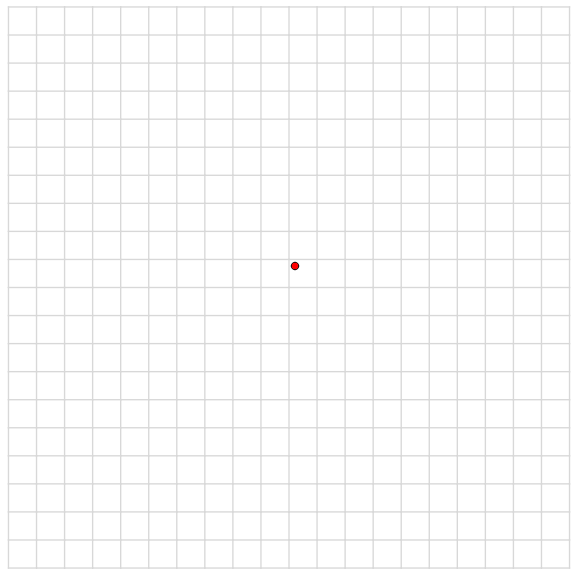##### Fig 1. Orthogonal network, 100 meters from node to node.

Procedure of calculating coverage using ‘pgrouting’.

In order to calculate a 500 meters coverage from red dot, you must follow the instructions:

• Duplicate the network and rename it as ‘network’..
• Create a table named ‘poi_tmp’, which will contain the geometries of the starting points.
• Modify ‘poi_tmp’ table, adding the fields(x,y,edge_id,side,fraction, newPoint).
 ```DO \$\$ #variable_conflict use_variable DECLARE dist_var INT; BEGIN dist_var:=500;DROP TABLE IF EXISTS "network"; CREATE TABLE IF NOT EXISTS "network" as (SELECT * FROM "orig_network"); drop table if exists poi_tmp; CREATE TABLE if not exists poi_tmp as (SELECT ST_Centroid(tmp."geom") the_geom,tmp."id" as pid from (SELECT * FROM "origin") tmp); ALTER TABLE poi_tmp ADD COLUMN     x FLOAT; ALTER TABLE poi_tmp ADD COLUMN     y FLOAT; ALTER TABLE poi_tmp ADD COLUMN     edge_id BIGINT; ALTER TABLE poi_tmp ADD COLUMN     side CHAR; ALTER TABLE poi_tmp ADD COLUMN     fraction FLOAT; ALTER TABLE poi_tmp ADD COLUMN     newPoint geometry;```
• Update the ‘edge_id’ field with the nearest section identifier.
• Update the ‘fraction’ field with the nearest section fraction.
 ```UPDATE "poi_tmp" set "edge_id"=nearest_section."tram_id" from (SELECT distinct on(Poi.pid) Poi.pid As Punt_id,Sg.id as Tram_id, ST_Distance(Sg.the_geom,Poi.the_geom) as dist FROM "network" as Sg,"poi_tmp" AS Poi ORDER BY Poi.pid, ST_Distance(Sg.the_geom,Poi.the_geom), Sg.id) nearest_section where "poi_tmp"."pid"=nearest_section."punt_id"; UPDATE "poi_tmp" SET fraction = ST_LineLocatePoint(e.the_geom, "poi_tmp".the_geom),newPoint = ST_LineInterpolatePoint(e.the_geom, ST_LineLocatePoint(e.the_geom, "poi_tmp".the_geom)) FROM "network" AS e WHERE "poi_tmp"."edge_id" = e.id;```
• Create ‘tbl_final_nodes_tmp’ table that will contain the ‘id’, aggregate costs and the starting node.
• Create ‘geo_final_nodes_tmp’ table that will contain the ‘tbl_final_nodes_tmp’ and their geometries.
 ```DROP FUNCTION IF EXISTS coverage(); DROP TABLE IF EXISTS tbl_final_nodes_tmp; CREATE TABLE IF NOT EXISTS tbl_final_nodes_tmp AS(SELECT node,agg_cost,start_vid FROM pgr_withPointsDD('SELECT id, source, target, cost, reverse_cost FROM "network" ORDER BY "network".id','SELECT pid, edge_id, fraction, side from "poi_tmp"',array(select "pid"*(-1) from "poi_tmp"),dist_var,driving_side := 'b',details := false)); DROP table if exists geo_final_nodes_tmp; CREATE TABLE IF NOT EXISTS geo_final_nodes_tmp as (select "orig_network_vertices_pgr".*,"tbl_final_nodes_tmp"."agg_cost", "tbl_final_nodes_tmp"."start_vid", dist_var from "orig_network_vertices_pgr","tbl_final_nodes_tmp" where "orig_network_vertices_pgr"."id" ="tbl_final_nodes_tmp"."node" order by "tbl_final_nodes_tmp"."start_vid" desc,"tbl_final_nodes_tmp"."agg_cost");```

After executing the previous code, you will obtaing the picture showed in Figure 2. The blue dot shows the projection of the starting point upon the nearest section, and this will be the starting point of every path calculated.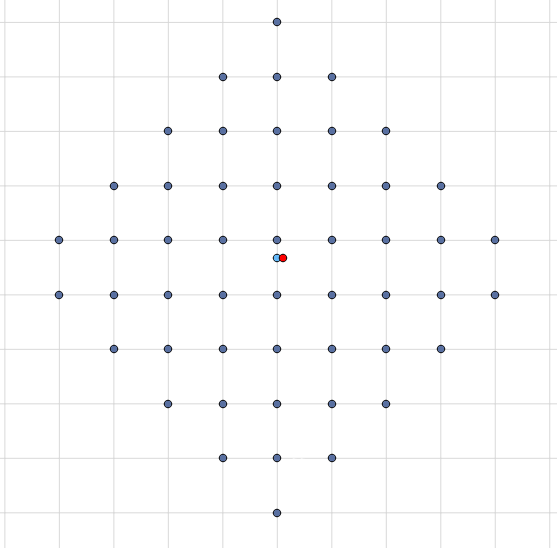##### Fig 2. Nodes conforming the coverage.

The ‘pgr_withPointsDD’ function, returns the nodes that conform the 500m coverage, but if you want to know where exactly finishes the coverage, you can’t.

In order to resolve this problem, continue reading:

• Create ‘final_sections_tmp’ table that will contain every section that will conform the coverage, including the final sections, but, as you may imagine, only a fraction of the every final section is valid.
 ```DROP table IF EXISTS final_sections_tmp; CREATE TABLE IF NOT EXISTS final_sections_tmp as (select "network"."id","network"."the_geom","geo_final_nodes_tmp"."id" as node,"geo_final_nodes_tmp"."agg_cost" as coste,(dist_var  "geo_final_nodes_tmp"."agg_cost") as falta,"geo_final_nodes_tmp"."start_vid" as id_punt,  (select case when (dist_var-"geo_final_nodes_tmp"."agg_cost") / ST_Length("network"."the_geom")<=1 then (dist_var-"geo_final_nodes_tmp"."agg_cost") / ST_Length("network"."the_geom") when (dist_var-      "geo_final_nodes_tmp"."agg_cost") / ST_Length("network"."the_geom")>1 then (1) end) as      fraccio from "network","geo_final_nodes_tmp" where ST_DWithin("geo_final_nodes_tmp"."the_geom", "network"."the_geom",1)=TRUE);```

You can see the result of executing the previous code in Figure 3.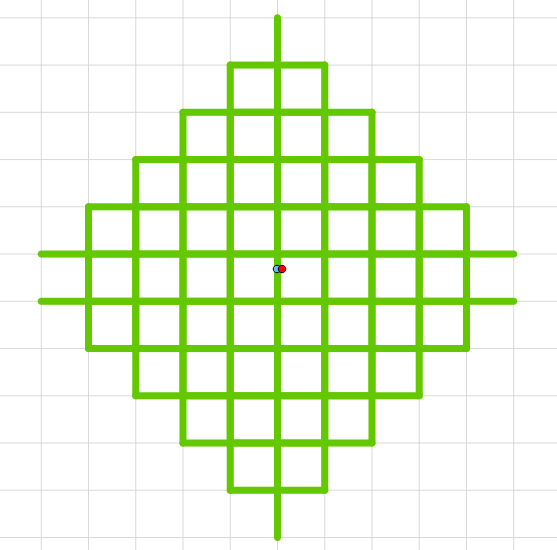##### Fig 3. Section conforming part of the 500m coverage.

In order to obtain the fractions of the final sections that complete the 500m coverage, below is a function written in ‘PL/pgSQL’, that does the necessary modifications.

• Create ‘Coverage’ function.
• Create ‘fraction_sections_raw’ table, that will contain complete and fractioned sections that conform the 500m coverage.
 ```CREATE OR REPLACE FUNCTION coverage() RETURNS SETOF final_sections_tmp AS \$BODY\$ DECLARE r final_sections_tmp%rowtype; m final_sections_tmp%rowtype; BEGIN DROP TABLE IF EXISTS fraction_sections_raw; CREATE TABLE fraction_sections_raw (the_geom geometry, punt_id bigint,id_tram bigint,fraccio FLOAT,node bigint,fraccio_inicial FLOAT,cost_invers FLOAT,cost_directe FLOAT,target bigint,radi_inic FLOAT); FOR r IN SELECT "final_sections_tmp".* FROM "final_sections_tmp" WHERE "final_sections_tmp"."id" not in (select "edge_id" from "poi_tmp") LOOP insert into fraction_sections_raw VALUES(ST_Line_Substring((r."the_geom"),case when (select ST_Line_Locate_Point((r."the_geom"),(select "geo_final_nodes_tmp"."the_geom" from "geo_final_nodes_tmp" where "geo_final_nodes_tmp"."id"=r."node" and "geo_final_nodes_tmp"."start_vid"=r."id_punt")))<0.001 then 0 else 1-r."fraccio" END, case when (select ST_Line_Locate_Point((r."the_geom"),(select "geo_final_nodes_tmp"."the_geom" from "geo_final_nodes_tmp" where "geo_final_nodes_tmp"."id"=r."node" and "geo_final_nodes_tmp"."start_vid"=r."id_punt")))<0.001 then r."fraccio" else 1 END),r."id_punt"*(-1),r."id",0,r."node",0,0,0,0); RETURN NEXT r; END LOOP; FOR m IN SELECT "final_sections_tmp".* FROM "final_sections_tmp" WHERE "final_sections_tmp"."id" in (select "edge_id" from "poi_tmp") LOOP insert into fraction_sections_raw VALUES(m."the_geom",m."id_punt"*(-1),m."id",0,m."node",0,0,0); RETURN NEXT m; END LOOP; RETURN; END \$BODY\$ LANGUAGE 'plpgsql' ; PERFORM "the_geom" FROM coverage();```

After that, is necessary to modify the ‘fraction_sections_raw’ table, in order to obtain the complete 500m coverage.

• Update ‘fraccio_inicial’ table with the calculated value.
• Update ‘cost_directe’ and ‘cost_invers’ fields with corresponding values.
• Update ‘fraccio’ field with the calculated values.
• Modify the geometry using the calulated ‘fraccio’ field.
 ```update "fraction_sections_raw" set "fraccio_inicial"="poi_tmp"."fraction" from "poi_tmp" where "id_tram"="edge_id"; update "fraction_sections_raw" set "cost_directe"="network"."cost", "target"="network"."target", "cost_invers"="network"."reverse_cost" from "network" where "id_tram"="id"; UPDATE "fraction_sections_raw" SET "fraccio"=((case when ("fraction_sections_raw"."fraccio_inicial" * ST_Length("fraction_sections_raw"."the_geom"))>dist_var then (dist_var/ST_Length("fraction_sections_raw"."the_geom")) else "fraction_sections_raw"."fraccio_inicial" end)+(case when ((1-"fraction_sections_raw"."fraccio_inicial") * ST_Length("fraction_sections_raw"."the_geom"))>dist_var then (dist_var/ST_Length("fraction_sections_raw"."the_geom")) else (1-"fraction_sections_raw"."fraccio_inicial") end)); update "fraction_sections_raw" set "the_geom"=final."the_geom"from(select distinct(ST_Line_Substring((m."the_geom"),(case when (select ST_Line_Locate_Point((m."the_geom"), (select "the_geom" from "geo_final_nodes_tmp" where "geo_final_nodes_tmp"."id"=m."node" and "geo_final_nodes_tmp"."start_vid" = m."punt_id"*-1)))<0.01 then 0 else 1-m."fraccio" END),(case when (select ST_Line_Locate_Point((m."the_geom") , (select "the_geom" from "geo_final_nodes_tmp" where "geo_final_nodes_tmp"."id"=m."node" and "geo_final_nodes_tmp"."start_vid" = m."punt_id"*-1)))<0.01 then m."fraccio" else 1 END)))  the_geom,m."id_tram"from "fraction_sections_raw" m where m."id_tram" in (select "edge_id" from "poi_tmp")) final where final."id_tram" ="fraction_sections_raw"."id_tram"; insert into "fraction_sections_raw" (select SX."the_geom",PI."pid" as punt_id,SX."id"as id_tram,999 as fraccio,SX."source" as node,PI."fraction" as fraccio_inicial , SX."cost",SX."reverse_cost" from "network" SX inner join (Select "edge_id","pid","fraction" from "poi_tmp") PI on SX."id"=PI."edge_id"); UPDATE "fraction_sections_raw" set "the_geom"=final."the_geom" from (select ST_Line_Substring((SXI."the_geom"),(case when (FT."fraccio_inicial" - (dist_var/ST_Length(SXI."the_geom")))>0 then (FT."fraccio_inicial"-(dist_var/ST_Length(SXI."the_geom"))) else 0 end),(case when (FT."fraccio_inicial" + (dist_var/ST_Length(SXI."the_geom")))<1 then (FT."fraccio_inicial"+(dist_var/ST_Length(SXI."the_geom"))) else 1 end)) as the_geom, FT."punt_id", FT."id_tram", FT."fraccio" from "fraction_sections_raw"FT inner join (select SX."the_geom" as the_geom, SX."id" as tram_xarxa from "orig_network" SX, "poi_tmp" PI where SX."id"=PI."edge_id") SXI on FT."id_tram"=SXI.tram_xarxa where FT."fraccio"=999) final where "fraction_sections_raw"."punt_id"=final."punt_id" and "fraction_sections_raw"."fraccio"=999;```
• Create ‘fractions_sections_tmp’ table that will contain every section that conform the 500m coverage.
• Create ‘driving_distance’ table that will contain the union of every section.
 ```DROP TABLE IF EXISTS fraction_sections_tmp; CREATE TABLE fraction_sections_tmp AS (select distinct(the_geom),punt_id,radi_inic from fraction_sections_raw); DROP TABLE IF EXISTS driving_distance; CREATE TABLE IF NOT EXISTS driving_distance AS (Select ST_Union(TOT.the_geom) the_geom, TOT."punt_id" from (select the_geom,punt_id,radi_inic from fraction_sections_tmp) TOT group by TOT."punt_id"); END \$\$;```

Once executed the previous code, you should have the same as you can see in the Figure 4.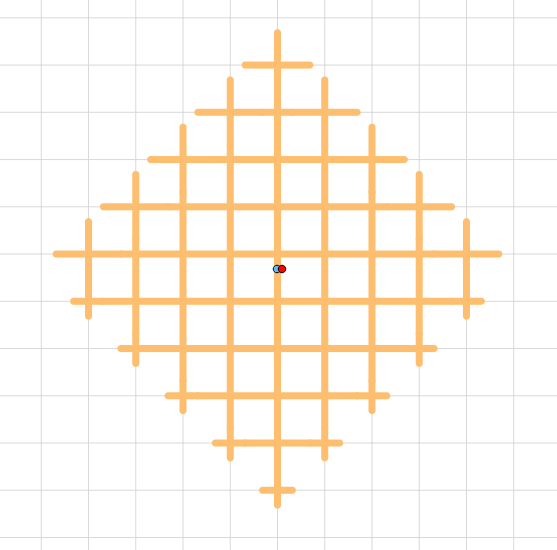##### Fig 4.  Final 500m coverage.

In Figure 4 you can see the final sections cutted where the 500m path finishes. You can compare with Figure 3 and see the differences.

Conclusions

As a final conclusion, we created a function that obtains the coverage through a network , from a given starting point and a given distance or cost function. Improving the result obtained using the functions of the ‘pgrouting’ library.

The designed function is applicable to any routing function in which starts from initial points, that can be outside of the network, to obtain the desired coverage.